# Conservation laws for a generalized Ito-type coupled KdV system

## Abstract

In this paper, the conservation laws for a generalized Ito-type coupled Korteweg-de Vries (KdV) system are constructed by increasing the order of the partial differential equations. The generalized Ito-type coupled KdV system is a third-order system of two partial differential equations and does not have a Lagrangian. The transformation $u={U}_{x}$, $v={V}_{x}$ converts the generalized Ito-type coupled KdV system into a system of fourth-order partial differential equations in U and V variables, which has a Lagrangian. Noether’s approach is then used to construct the conservation laws. Finally, the conservation laws are expressed in the original variables u and v. Some local and infinitely many nonlocal conserved quantities are found for the generalized Ito-typed coupled KdV system.

## 1 Introduction

In this paper, we consider the generalized Ito-type coupled Korteweg-de Vries (KdV) system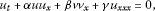(1a)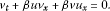(1b)

It is well known that coupled nonlinear systems in which a KdV structure is embedded occur naturally in shallow water wave problems [2, 3]. When $\alpha =-6$, $\beta =-2$ and $\gamma =-1$, the system (1a) and (1b) is called Ito’s system and it describes the interaction process of two internal long waves [4, 5]. It should be noted that in the absence of the effect of v, the system (1a) and (1b) reduces to the ordinary KdV equation. In  it has been shown that this Ito’s system can be a member of a bi-Hamiltonian integrable hierarchy. The numerical methods for this system are very limited . However, Xu and Shu  developed local discontinuous Galerkin methods for Ito’s system and proved the ${L}^{2}$ stability of these methods and, as a result, showed some good numerical results. Recently, in , the generalized Ito-type coupled KdV system (1a) and (1b) was constructed as a multi-symplectic Hamiltonian partial differential equation by introducing some new variables, and multi-symplectic numerical methods were applied to investigate this system.

In this paper, we derive conservation laws for the generalized Ito-type coupled KdV system (1a) and (1b). It is well known that the conservation laws play a central role in the solution and reduction of partial differential equations. Conservation laws are mathematical expressions of the physical laws, such as conservation of energy, mass, momentum and so on. In the literature, conservation laws have been extensively used in studying the existence, uniqueness and stability of solutions of nonlinear partial differential equations (see, for example, ). Conservation laws have also been applied in the development and use of numerical methods (see for example, [10, 11]). Recently, conserved vectors associated with Lie point symmetries have been used to find exact solutions (by exploiting a double reduction method) of some classical partial differential equations . Thus, it is important to derive all the conservation laws for a given differential equation.

For variational problems, the celebrated Noether theorem  provides an elegant way to construct conservation laws. In fact, it gives an explicit formula for determining a conservation law once a Noether symmetry associated with a Lagrangian is known for an Euler-Lagrange equation. Thus, the knowledge of a Lagrangian is essential in this case. However, there are differential equations, such as scalar evolution differential equations, which do not have a Lagrangian. In such cases, several methods  have been developed by researchers about the construction of conserved quantities. Comparison of several different methods for computing conservation laws can be found in [21, 27].

## 2 Conservation laws for the Ito-type coupled KdV system

In this section, we construct conservation laws for the Ito-type KdV system (1a) and (1b). We note that this system does not have a Lagrangian. However, we can put the system into a variational form by letting $u={U}_{x}$, $v={V}_{x}$. Then the Ito-type coupled KdV system (1a) and (1b) transforms into a fourth-order system, viz.,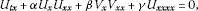(2a)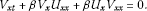(2b)

It can be readily verified that L given by

$L=\frac{1}{2}\left\{\gamma {U}_{xx}^{2}-\frac{\alpha }{3}{U}_{x}^{3}-\beta {U}_{x}{V}_{x}^{2}-{U}_{t}{U}_{x}-{V}_{t}{V}_{x}\right\}$
(3)

is a Lagrangian for the system (2a) and (2b). This is because L satisfies

$\frac{\delta L}{\delta U}=0\phantom{\rule{1em}{0ex}}\text{and}\phantom{\rule{1em}{0ex}}\frac{\delta L}{\delta V}=0,$
(4)

where $\delta /\delta U$ and $\delta /\delta V$ are the Euler-Lagrange operators defined by

$\frac{\delta }{\delta U}=\frac{\partial }{\partial U}-{D}_{t}\frac{\partial }{\partial {U}_{t}}-{D}_{x}\frac{\partial }{\partial {U}_{x}}+{D}_{t}^{2}\frac{\partial }{\partial {U}_{tt}}+{D}_{x}^{2}\frac{\partial }{\partial {U}_{xx}}+{D}_{x}{D}_{t}\frac{\partial }{\partial {U}_{tx}}-\cdots$
(5)

and

$\frac{\delta }{\delta V}=\frac{\partial }{\partial V}-{D}_{t}\frac{\partial }{\partial {V}_{t}}-{D}_{x}\frac{\partial }{\partial {V}_{x}}+{D}_{t}^{2}\frac{\partial }{\partial {V}_{tt}}+{D}_{x}^{2}\frac{\partial }{\partial {V}_{xx}}+{D}_{x}{D}_{t}\frac{\partial }{\partial {V}_{tx}}-\cdots .$
(6)

Consider the vector field

$X={\xi }^{1}\left(t,x,U,V\right)\frac{\partial }{\partial t}+{\xi }^{2}\left(t,x,U,V\right)\frac{\partial }{\partial x}+{\eta }^{1}\left(t,x,U,V\right)\frac{\partial }{\partial U}+{\eta }^{2}\left(t,x,U,V\right)\frac{\partial }{\partial V},$
(7)

which has the second extension

$\begin{array}{rcl}{X}^{\left[2\right]}& =& {\xi }^{1}\left(t,x,U,V\right)\frac{\partial }{\partial t}+{\xi }^{2}\left(t,x,U,V\right)\frac{\partial }{\partial x}+{\eta }^{1}\left(t,x,U,V\right)\frac{\partial }{\partial U}\\ +{\eta }^{2}\left(t,x,U,V\right)\frac{\partial }{\partial V}+{\zeta }_{t}^{1}\frac{\partial }{\partial {U}_{t}}+{\zeta }_{t}^{2}\frac{\partial }{\partial {V}_{t}}+{\zeta }_{x}^{1}\frac{\partial }{\partial {U}_{x}}+{\zeta }_{x}^{2}\frac{\partial }{\partial {V}_{x}}+\cdots ,\end{array}$
(8)

where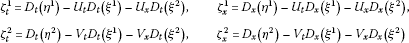and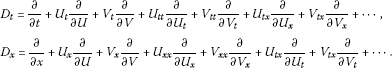We recall that the vector field X, of the form (7), is called a Noether point symmetry generator corresponding to the Lagrangian L if there exists gauge functions ${B}^{1}\left(t,x,U,V\right)$ and ${B}^{2}\left(t,x,U,V\right)$ such that

${X}^{\left[2\right]}\left(L\right)+\left\{{D}_{t}\left({\xi }^{1}\right)+{D}_{x}\left({\xi }^{2}\right)\right\}L={D}_{t}\left({B}^{1}\right)+{D}_{x}\left({B}^{2}\right).$
(9)

The insertion of L from (3) into Eq. (9) yields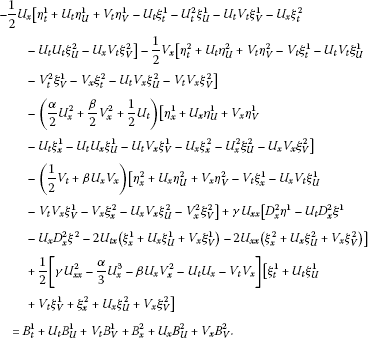(10)

The analysis of Eq. (10) prompts the following two cases.

### 2.1 Case 1 $\alpha \ne \beta$

In this case, the splitting of (10) with respect to different combinations of the derivatives of U and V gives an overdetermined system of linear partial differential equations for ${\xi }^{1}$, ${\xi }^{2}$, ${\eta }^{1}$, ${\eta }^{2}$, ${B}^{1}$ and ${B}^{2}$. Solving this system yields the following Noether symmetries and gauge terms: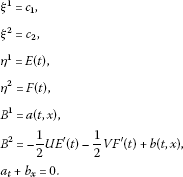(11)

The above results will now be used to find the components of the conserved vectors for the second-order Lagrangian L. Here we can choose $a=0$, $b=0$ as they contribute to the trivial part of the conserved vector. We recall that the conserved vectors for the second-order Lagrangian L are given by [15, 27]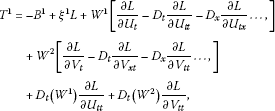(12)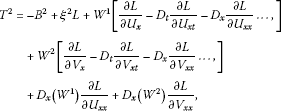(13)

where ${W}^{1}={\eta }^{1}-{U}_{t}{\xi }^{1}-{U}_{x}{\xi }^{2}$ and ${W}^{2}={\eta }^{2}-{V}_{t}{\xi }^{1}-{V}_{x}{\xi }^{2}$ are the Lie characteristic functions. Thus, using (12) and (13) together with (11) and $u={U}_{x}$, $v={V}_{x}$ yields the following independent conserved vectors for our system (1a) and (1b):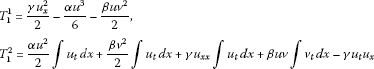(14)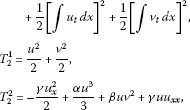(15)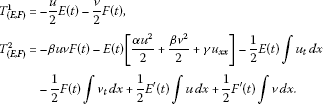(16)

It should be noted that the conserved vectors (14) and (16) are nonlocal conserved vectors and (15) is a local conserved vector for the system (1a) and (1b). We now extract two special cases from the conserved vector (16) by letting $E\left(t\right)=1$ and $F\left(t\right)=0$, which gives a nonlocal conserved vector

$\begin{array}{r}{T}_{3}^{1}=-\frac{u}{2},\\ {T}_{3}^{2}=-\frac{\alpha {u}^{2}}{2}-\frac{\beta {v}^{2}}{2}-\gamma {u}_{xx}-\frac{1}{2}\int {u}_{t}\phantom{\rule{0.2em}{0ex}}dx,\end{array}$
(17)

and by choosing $E\left(t\right)=0$ and $F\left(t\right)=1$, which gives a nonlocal conserved vector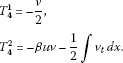We note that since the functions $E\left(t\right)$ and $F\left(t\right)$ are arbitrary, one obtains infinitely many nonlocal conservation laws for the system (1a) and (1b). It should also be noted that for the special values of α, β and γ, namely, $\alpha =-6$, $\beta =-2$ and $\gamma =-1$, we retrieve the three constants of the motion ${F}_{0}$, ${F}_{1}$ and ${F}_{2}$ obtained in .

### 2.2 Case 2 $\alpha =\beta$

In this case, we obtain the following Noether symmetries and gauge terms: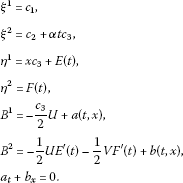(18)

Again, we can set $a=0$, $b=0$ as they contribute to the trivial part of the conserved vector. The independent conserved vectors for the system (1a) and (1b) in this case are as follows: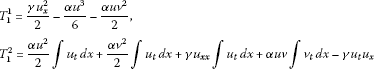(19)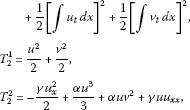(20)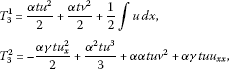(21)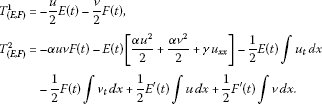(22)

Note that the conserved vectors (19), (21) and (22) are nonlocal conserved vectors whereas the conserved vector (20) is a local conserved vector for the system (1a) and (1b). The conserved vector (22), for $E\left(t\right)=1$ and $F\left(t\right)=0$, gives a nonlocal conserved vector

$\begin{array}{r}{T}_{4}^{1}=-\frac{u}{2},\\ {T}_{4}^{2}=-\frac{\alpha {u}^{2}}{2}-\frac{\alpha {v}^{2}}{2}-\gamma {u}_{xx}-\frac{1}{2}\int {u}_{t}\phantom{\rule{0.2em}{0ex}}dx,\end{array}$
(23)

and for $E\left(t\right)=0$ and $F\left(t\right)=1$, it gives a nonlocal conserved vector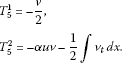We see that for the arbitrary values of $E\left(t\right)$ and $F\left(t\right)$, infinitely many nonlocal conservation laws exist for the system (1a) and (1b).

## 3 Concluding remarks

In this paper, we studied the generalized Ito-type coupled Korteweg-de Vries system (1a) and (1b). This system does not have a Lagrangian. In order to apply Noether’s theorem, we transformed the system into the fourth-order system (2a) and (2b), which admitted a standard Lagrangian (3). Then Noether’s approach was used to derive the conservation laws in U and V variables. Finally, by reverting back to our original variables u and v, we obtained the conservation laws for the third-order generalized Ito-type coupled KdV system (1a) and (1b). The conservation laws for the generalized Ito-type coupled KdV system consisted of some local and infinite number of nonlocal conserved vectors.

## References

1. Chen Y, Song S, Zhu H: Multi-symplectic methods for the Ito-type coupled KdV equation. Appl. Math. Comput. 2012, 218: 5552-5561. 10.1016/j.amc.2011.11.045

2. Whitham GB: Linear and Nonlinear Waves. Wiley, New York; 1974.

3. Xu Y, Shu C-W: Local discontinuous Galerkin methods for the Kuramoto-Sivashinsky equations and the Ito-type coupled KdV equations. Comput. Methods Appl. Mech. Eng. 2006, 195: 3430-3447. 10.1016/j.cma.2005.06.021

4. Ito M: Symmetries and conservation laws of a coupled nonlinear wave equation. Phys. Lett. A 1982, 91: 335-338. 10.1016/0375-9601(82)90426-1

5. Guha-Roy C: Solution of coupled KdV-type equations. Int. J. Theor. Phys. 1990, 29(8):863-866. 10.1007/BF00675103

6. Kupershmidt BA: A coupled Korteweg-de Vries equation with dispersion. J. Phys. A, Math. Gen. 1985, 18: 571-573. 10.1088/0305-4470/18/10/003

7. Lax PD: Integrals of nonlinear equations of evolution and solitary waves. Commun. Pure Appl. Math. 1968, 21: 467-490. 10.1002/cpa.3160210503

8. Benjamin TB: The stability of solitary waves. Proc. R. Soc. Lond. Ser. A, Math. Phys. Sci. 1972, 328: 153-183. 10.1098/rspa.1972.0074

9. Knops RJ, Stuart CA: Quasiconvexity and uniqueness of equilibrium solutions in nonlinear elasticity. Arch. Ration. Mech. Anal. 1984, 86: 234-249.

10. LeVeque RJ: Numerical Methods for Conservation Laws. Birkhäuser, Basel; 1992.

11. Godlewski E, Raviart P-A: Numerical Approximation of Hyperbolic Systems of Conservation Laws. Springer, Berlin; 1996.

12. Sjöberg A: Double reduction of PDEs from the association of symmetries with conservation laws with applications. Appl. Math. Comput. 2007, 184: 608-616. 10.1016/j.amc.2006.06.059

13. Bokhari AH, Al-Dweik AJ, Kara AH, Mahomed FM, Zaman FD:Double reduction of a nonlinear $\left(2+1\right)$ wave equation via conservation laws. Commun. Nonlinear Sci. Numer. Simul. 2011, 16: 1244-1253. 10.1016/j.cnsns.2010.07.007

14. Caraffini GL, Galvani M: Symmetries and exact solutions via conservation laws for some partial differential equations of mathematical physics. Appl. Math. Comput. 2012, 219: 1474-1484. doi:10.1016/j.amc.2012.07.050 10.1016/j.amc.2012.07.050

15. Noether E: Invariante variationsprobleme. Nachr. Konig. Gesell. Wissen., Gottingen, Math.-Phys. Kl. 1918, 2: 235-257. English translation in Transp. Theory and Stat. Phys. 1(3), 186-207 (1971)

16. Laplace PS 1. In Traité de Mécanique Céleste. Duprat, Paris; 1798. English translation: Celestial Mechanics, New York (1966)

17. Steudel H: Uber die Zuordnung zwischen Invarianzeigenschaften und Erhaltungssatzen. Z. Naturforsch. 1962, 17: 129-132.

18. Olver PJ: Application of Lie Groups to Differential Equations. 2nd edition. Springer, New York; 1993.

19. Anco SC, Bluman GW: Direct construction method for conservation laws of partial differential equations. Part I: examples of conservation law classifications. Eur. J. Appl. Math. 2002, 13: 545-566.

20. Anco SC, Bluman GW: Direct construction method for conservation laws of partial differential equations. part II: general treatment. Eur. J. Appl. Math. 2002, 13: 567-585.

21. Wolf T: A comparison of four approaches to the calculation of conservation laws. Eur. J. Appl. Math. 2002, 13: 129-152.

22. Kara AH, Mahomed FM: Relationship between symmetries and conservation laws. Int. J. Theor. Phys. 2000, 39: 23-40. 10.1023/A:1003686831523

23. Kara AH, Mahomed FM: Noether-type symmetries and conservation laws via partial Lagrangians. Nonlinear Dyn. 2006, 45: 367-383. 10.1007/s11071-005-9013-9

24. Atherton RW, Homsy GM: On the existence and formulation of variational principles for nonlinear differential equations. Stud. Appl. Math. 1975, 54: 31-60.

25. Ibrahimov NH, Kolsrud T: Lagrangian approach to evolution equations: symmetries and conservation laws. Nonlinear Dyn. 2004, 36(1):29-40.

26. Ibragimov NH: A new conservation theorem. J. Math. Anal. Appl. 2007, 333: 311-328. 10.1016/j.jmaa.2006.10.078

27. Naz R, Mason DP, Mahomed FM: Conservation laws and conserved quantities for laminar two-dimensional and radial jets. Nonlinear Anal., Real World Appl. 2009, 10: 2641-2651. 10.1016/j.nonrwa.2008.07.003

## Acknowledgements

This paper is dedicated to Prof. Ravi P. Agarwal on his 65th birth anniversary. BM and CMK would like to thank the Organizing Committee of ‘International Conference on Applied Analysis and Algebra’, (ICAAA 2012) Conference for their kind hospitality during the conference. BM also thanks the Faculty Research Committee of FAST, North-West University, and ETM thanks SANHARP for financial support.

## Author information

Authors

### Corresponding author

Correspondence to Ben Muatjetjeja.

### Competing interests

The authors declare that they have no competing interests.

### Authors’ contributions

ETM, BM and CMK worked together in the derivation of the mathematical results. All authors read and approved the final manuscript.

## Rights and permissions

Reprints and Permissions

Mogorosi, E.T., Muatjetjeja, B. & Khalique, C.M. Conservation laws for a generalized Ito-type coupled KdV system. Bound Value Probl 2012, 150 (2012). https://doi.org/10.1186/1687-2770-2012-150

• Accepted:

• Published:

• DOI: https://doi.org/10.1186/1687-2770-2012-150

### Keywords

• Discontinuous Galerkin Method
• Water Wave Problem
• Local Discontinuous Galerkin Method
• Gauge Term
• Trivial Part